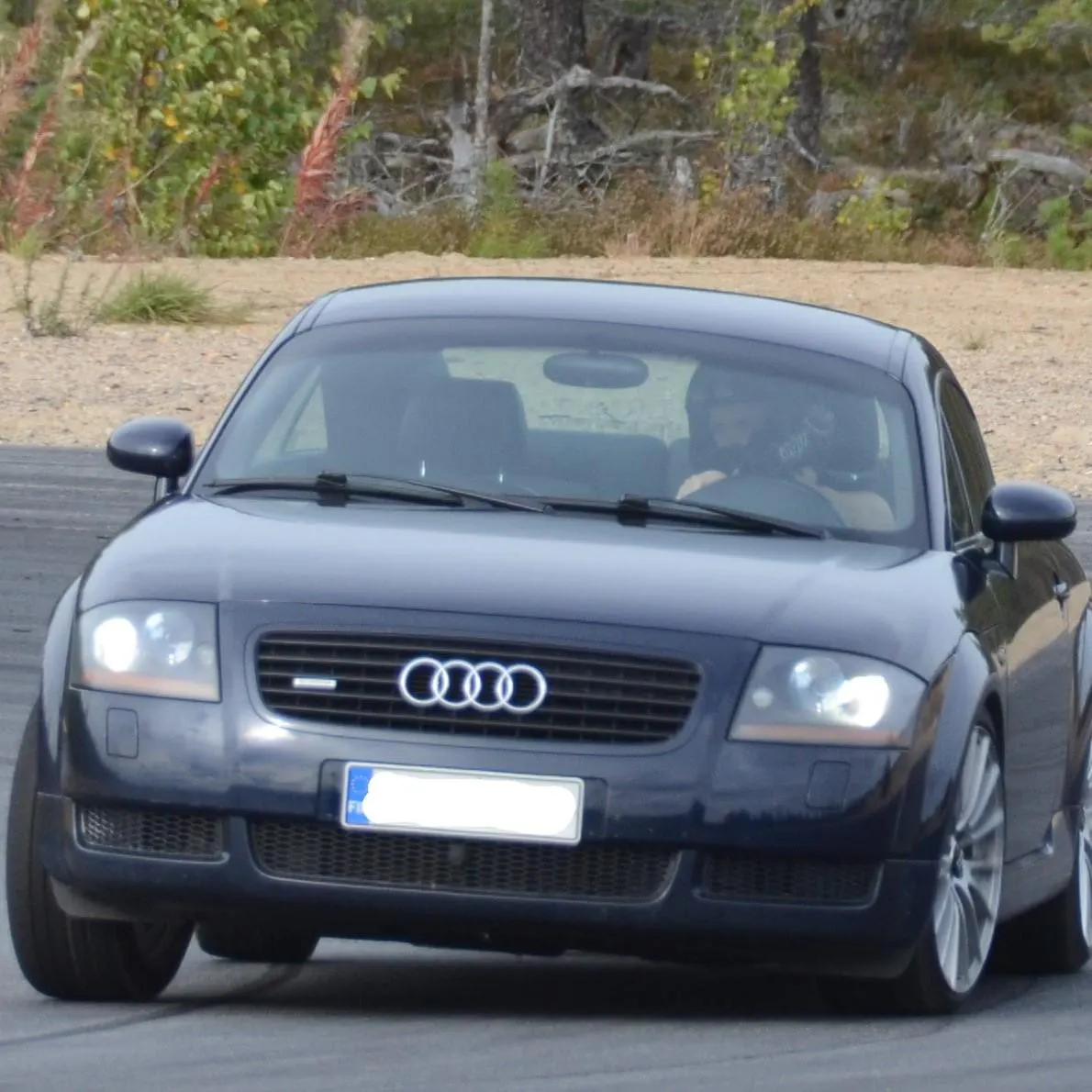# Digital Speedometer to Car's Instrument Cluster via CAN Bus

Implementing Arduino CAN bus shield and digital speedometer to car's LCD-display in instrument cluster.

IntermediateFull instructions providedOver 2 days18,179## Things used in this project

### Hardware componentsArduino Nano R3
×1
 MCP2515
×1
 NXP TJA1055
×1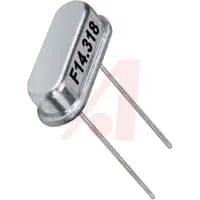16 MHz Crystal
×1Resistor 10k ohm
×1Resistor 1k ohm
×1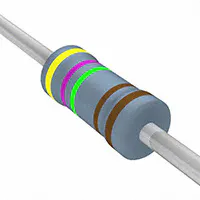Resistor 4.75k ohm R3, R4 Bus Termination Resistors
×2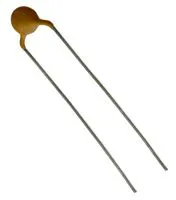Ceramic Disc Capacitor, 150 pF Bus capacitors for EMI improvements
×2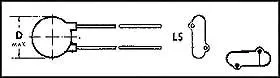Ceramic Disc Capacitor, 18 pF Capacitors for 16MHz crystal oscillator
×2
 Screw Block Terminal 5-R3.5
×1

### Software apps and online servicesArduino IDE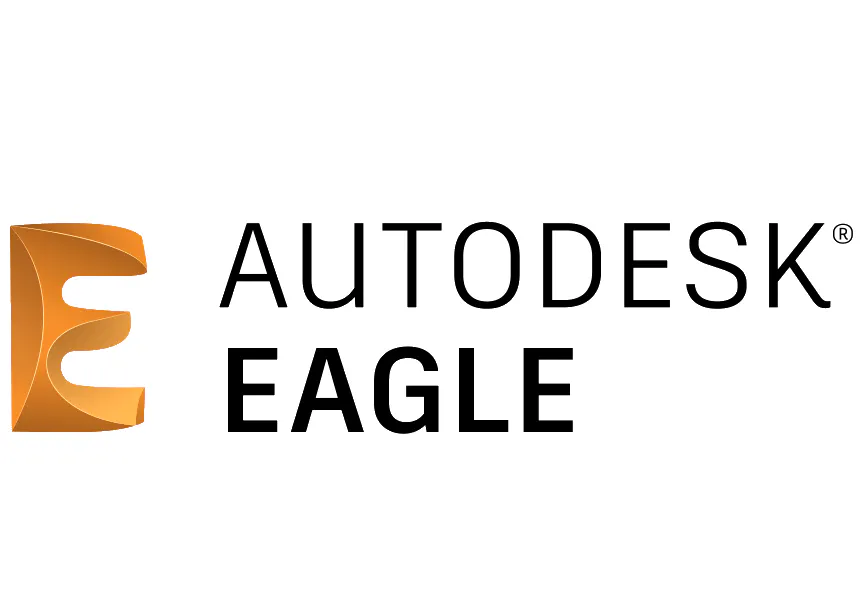Autodesk EAGLE

## Schematics

### Board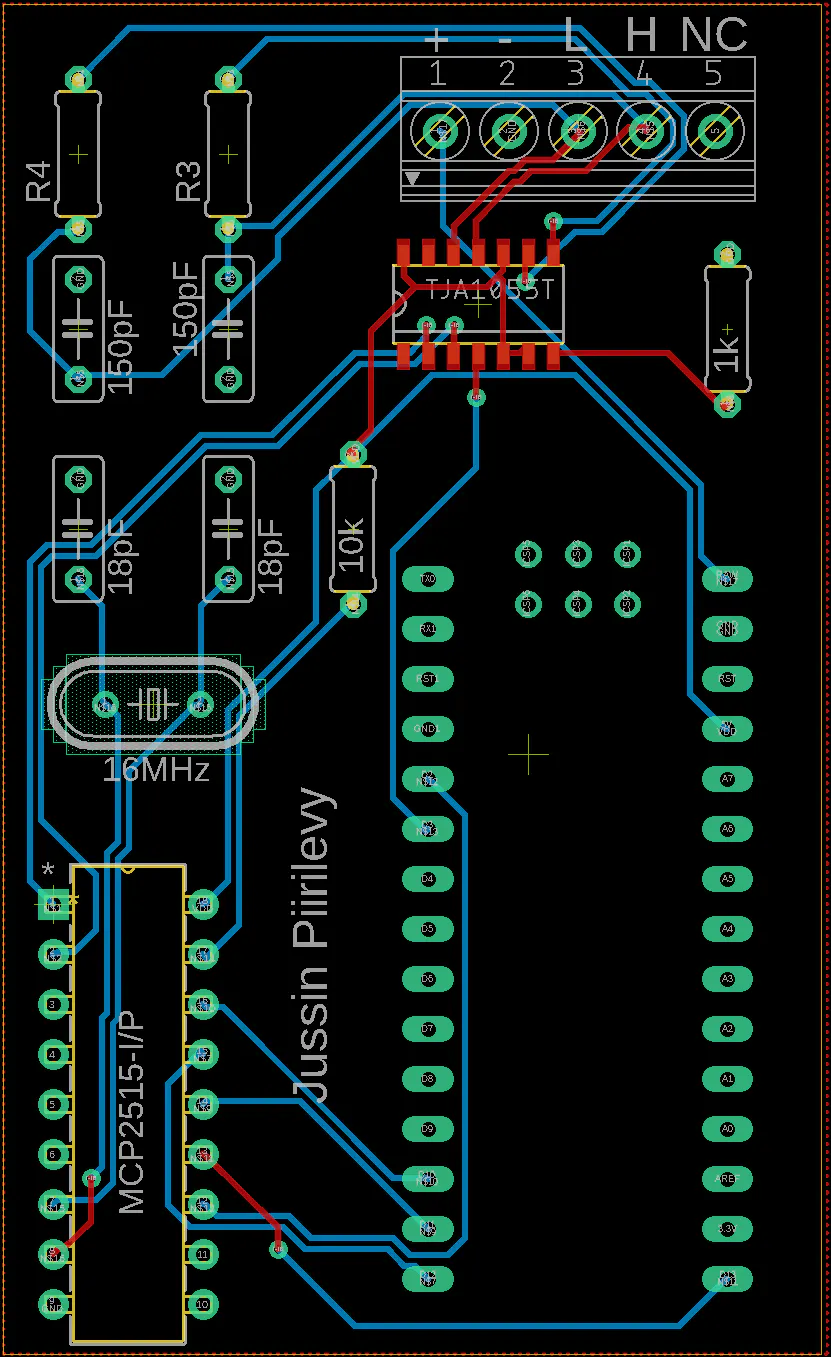### Schematics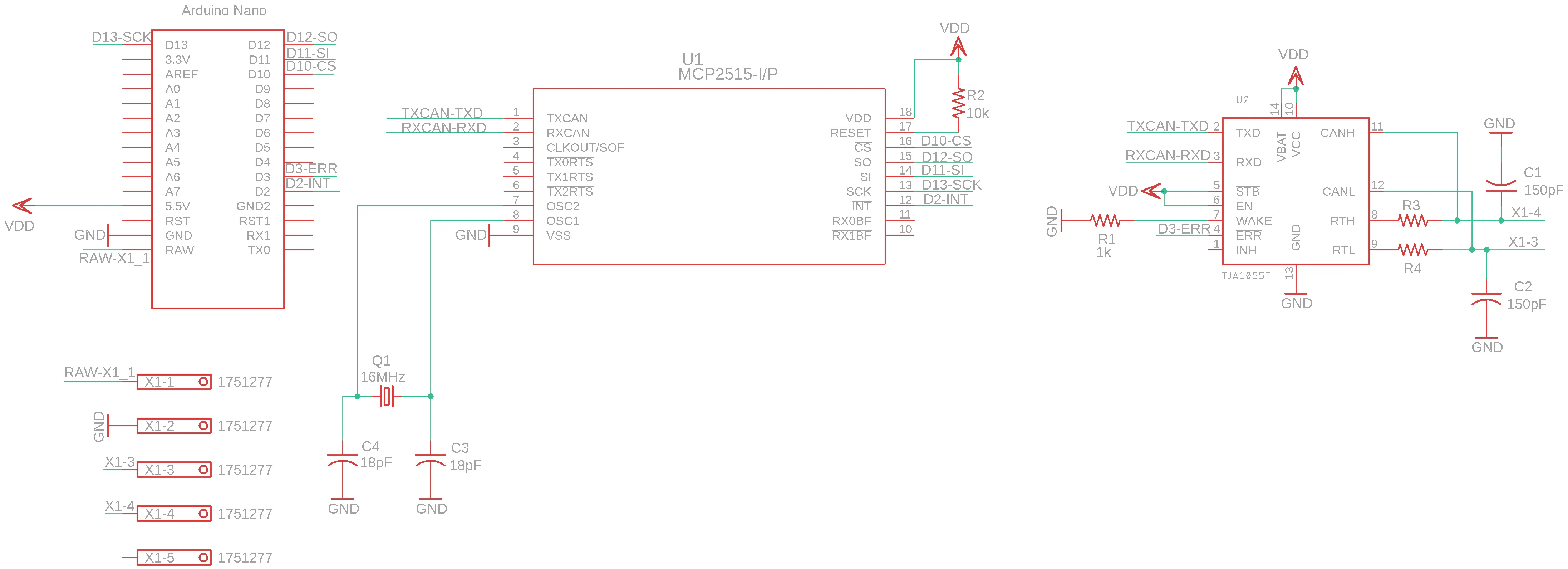## Code

### Can Bus Speedometer

Arduino
Code for the setup with CAN bus compatible radio (Chorus)
```#include <SPI.h>
#include <mcp2515.h>

struct can_frame canMsg, canMsg1, canMsg2;

int velocity,lv,uv = 0;
const int INTERVAL = 800; //  ms
const int REFRESH_RATE = 20; // ms
bool init_state = true;

const uint8_t ASCII  = {0x30,0x31,0x32,0x33,0x34,0x35,0x36,0x37,0x38,0x39}; // ASCII for numbers 0..9
const uint8_t SPACE = 0x20;
const uint8_t KMH_MESSAGE  = {SPACE, SPACE, 0xB, 0xD, 0x2f, 0x8, SPACE, SPACE}; //ASCII for '  km/h  '

const uint8_t AUDI_TTe  = {65, 117, 4, 9, 32, 84, 84, 5}; // "Audi TTe"
const uint8_t QUATTRO  = {113, 117, 0x01, 116, 116, 114, 0xF, 32}; // "quattro"
const uint32_t MASK = 0xD440000; // Mask for id 0x351 = 0b 0110 1010 00 1 <=> 0xD440000 = 0b 0110 1010 00 1 0000 0000 0000 0000 00 . Extended from 11 to 29 bits
const int SPEED_ID = 0x351;

MCP2515 mcp2515(10);

void setup() {

canMsg1.can_id  = 0x261; // Message ID for the first row of the DIS
canMsg1.can_dlc = 8;

canMsg2.can_id  = 0x263; // Message ID for the second row of the DIS
canMsg2.can_dlc = 8;
for(int j=0; j<8; j++){
canMsg2.data[j] = KMH_MESSAGE[j];
}

mcp2515.setBitrate(CAN_100KBPS, MCP_16MHZ);
mcp2515.setNormalMode();

}

void clear(){
for(int i=0; i<8; i++){
canMsg1.data[i] = SPACE; // Initialising the speed message (first row of
canMsg2.data[i] = KMH_MESSAGE[i];;                     // DIS) with SPACES
}
}

if(canMsg.can_id == id){
return true;
}
return false;
}

}

void audi_tt(){

for(int i=0; i<8; i++){
canMsg1.data[i] = AUDI_TTe[i];
canMsg2.data[i] = QUATTRO[i];
}
for(int j=0; j<3000/REFRESH_RATE;j++){
mcp2515.sendMessage(&canMsg1);
mcp2515.sendMessage(&canMsg2);
delay(REFRESH_RATE);
}

}

void loop() {

if(init_state){
audi_tt();
init_state = false;
clear();
}

clear();
lv = canMsg.data;
uv = canMsg.data;
velocity = ((uv<<8)+lv-1)/200;
velocity = round(velocity);

if(velocity < 10){
canMsg1.data = ASCII[velocity];
}

else if(velocity < 100){
canMsg1.data = ASCII[velocity/10]; // Splitting integer to single digits
canMsg1.data = ASCII[velocity%10];
}
else{
canMsg1.data = ASCII[velocity/100];
canMsg1.data = ASCII[(velocity/10)%10];
canMsg1.data = ASCII[velocity%10];

}

int i = 0;
while(true){
if(i >= INTERVAL){
return;
}

else if(i % REFRESH_RATE == 0){
mcp2515.sendMessage(&canMsg1);
mcp2515.sendMessage(&canMsg2);
}
i++;
delay(1);
}

}
// The car radio sends it own radio station data every 0,8s to
// the instrument cluster, so I have to send my data with much
// higher rate, so the display won't start to blink
}
```

### GALA Speedometer

Arduino
Code for the setup with Concert-radio
```/*
Digital speedometer to Audi Driver Information System

Reads vehicle speed from Audi Concert Gala-wire (
Sends information to instrument cluster via Audi concert pins)

*/
#define pulse_ip 2
#define enable 6
#define sck 7
#define sda 8

unsigned long ontime,offtime, period;
float freq = 0;
int final_speed = 0;
float speed_ = 0;
float circumference = 0.63719; // in meters
uint8_t command_byte = 0x1c;
uint8_t message , checksum;

// numbers from 0 to 9 in ASCII-form
uint8_t ASCII  = {0x30,0x31,0x32,0x33,0x34,0x35,0x36,0x37,0x38,0x39};

uint8_t KMH  = {0x20,0x4B,0x4D, 0x2F,0x48}; // ASCII for: ' km/h'
uint8_t SPACE = 0x20; // ASCII for: ' '

// the setup function runs once when you press reset or power the board
void setup() {

pinMode(pulse_ip, INPUT); // pin for vehicle speed signal (GALA)

pinMode(enable, OUTPUT);
pinMode(sck, OUTPUT);
pinMode(sda, OUTPUT);
}

// the loop function runs over and over again forever
void loop() {

ontime = pulseIn(pulse_ip,HIGH);
offtime = pulseIn(pulse_ip,LOW);
period = ontime+offtime;
freq = 1000000.0/(period*2); // 2 periods per one wheel rotation
speed_ = freq*circumference*3.6;
speed_ = round(speed_);
final_speed = speed_;

message = command_byte;

message = SPACE; // For alignment
message = SPACE;

if(final_speed < 10){
message = ASCII[final_speed];
message = SPACE;
message = SPACE;
}

else if(final_speed < 100){
message = ASCII[final_speed / 10];
message = ASCII[final_speed % 10];
message = SPACE;
}

else{
message = ASCII[final_speed / 100];
message = ASCII[final_speed / 10];
message = ASCII[final_speed % 10];
}

for(int i = 6; i < 11; i++){ // for alignment
message[i] = SPACE;
}

message = KMH;
message = KMH;
message = KMH;
message = KMH;

message = SPACE;

checksum = 0;
for(int j = 0; j < 17; j++){
checksum += message[j];
}

checksum ^= 0xff;
message = checksum;

digitalWrite(enable, HIGH);

// Sending the data
for(int i = 0; i < 18; i++){
uint8_t data = message[i];

for(int k = 0; k < 8; k++){

if(data & 0x80){
digitalWrite(sda, HIGH);
}
else{
digitalWrite(sda, LOW);
}
digitalWrite(sck, LOW);
digitalWrite(sck, HIGH);
data <<=1; //Shifting 1 left
}
}
digitalWrite(enable, LOW);
delay(500);
}
```

## Credits

### Jussi Ristiniemi

0 projects • 6 followers